×#### Thank you for registering.

One of our academic counsellors will contact you within 1 working day.

Click to Chat

1800-1023-196

+91-120-4616500

CART 0

• 0

MY CART (5)

Use Coupon: CART20 and get 20% off on all online Study Material

ITEM
DETAILS
MRP
DISCOUNT
FINAL PRICE
Total Price: Rs.

There are no items in this cart.
Continue Shopping• Complete JEE Main/Advanced Course and Test Series
• OFFERED PRICE: Rs. 15,900
• View Details

```Chapter 13 Simple Interest

Question: 1

Find the simple interest , when :

Solution:

(i) . Principal = Rs . 2000 , Rate of interest = 5% per annum , and Time = 5 years(ii) . Principal = Rs . 500 , Rate of interest = 12.5% per annum , and Time = 4 years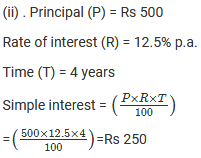(iii) . Principal = Rs . 4500 , Rate of interest = 4% per annum , and Time = 6 months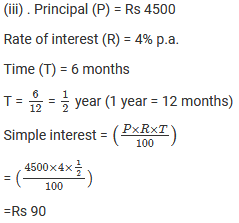(iv) . Principal =Rs . 12000 , Rate of interest = 18% per annum , and Time = 4 months(v) . Principal = Rs . 1000 , Rate of interest = 10% per annum , and Time = 73 days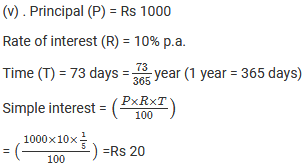Question: 2

Find the interest on Rs . 500 for a period of 4 years at the rate of 8% per annum . Also , find the amount to be paid at the end of the period .

Solution: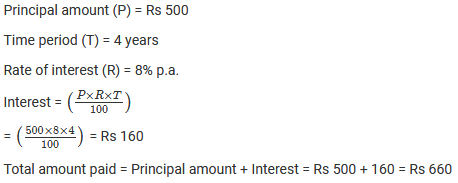Question: 3

A sum of Rs . 400 is lent at the rate of 5% per annum . Find the interest at the end of 2 years .

Solution:Question: 4

A sum of Rs . 400 is lent for 3 years at the rate of 6% per annum . Find the interest .

Solution: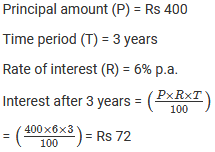Question: 5

A person deposits Rs . 25000 in a firm who pays an interest at the rate of 20 % per annum . Calculate the income he gets from it annually .

Solution:Question: 6

A man borrowed Rs . 8000 from a bank at 8% per annum . Find the amount he has to pay after 412 years .

Solution:Question: 7

Rakesh lent out Rs . 8000 for 5 years at 15 % per annum and borrowed Rs . 6000 for 3 years at 12% per annum . How much did he gain or lose ?

Solution:Question: 8

Anita deposits Rs . 1000 in a savings bank account . The bank pays interest at the rate of 5 % per annum . What amount can Anita get after 1 year ?

Solution: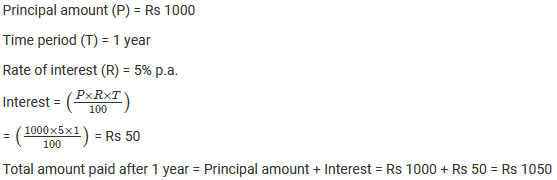Question: 9

Nalini borrowed Rs . 550 from her friend at 8% per annum . She returned the amount after six months . How much did she pay ?

Solution: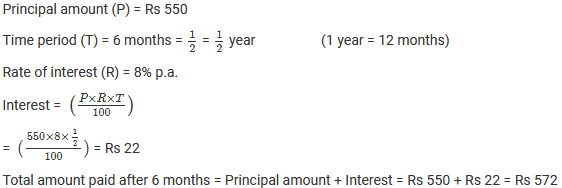Question: 10

Rohit borrowed Rs . 60000 from a bank at 9% per annum for 2 years . He lent this sum of money to Rohan at 10% per annum for 2 years . How much did Rohit earn from this transaction ?

Solution: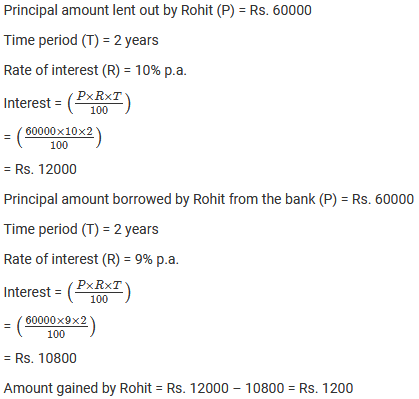Question: 11

Romesh borrowed Rs . 2000 at 2% per annum and Rs . 1000 at 5% per annum . He cleared his debt after 2 years by giving Rs . 2800 and a watch . What is the cost of watch ?

Solution: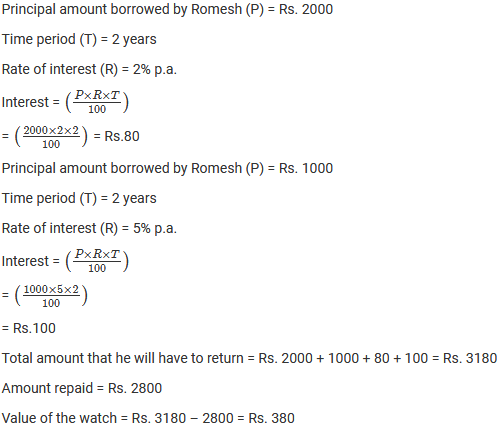Question: 12

Mr.Garg lent Rs . 15000 to his friend . He charged 15% per annum on Rs. 12500 and 18% on the rest . How much interest does he earn in 3 years ?

Solution: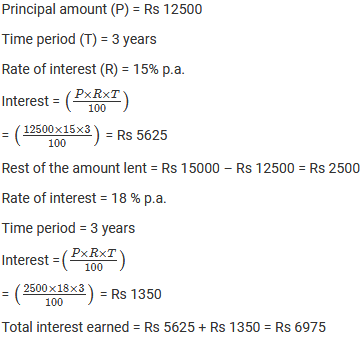Question: 13

Shikha deposited Rs . 2000 in a bank which pays 6% simple interest . She withdrew Rs . 700 at the end of first year .What will be her balance after 3 years ?

Solution:Question: 14

Reema took a loan of Rs . 8000 from a money lender , who charged interest at the rate of 18% per annum . After 2 years , Reema paid him Rs . 10400 and wrist watch to clear the debt . What is the price of the watch ?

Solution:Question: 15

Mr. Sharma deposited Rs. 20000 as a fixed deposit in a bank at 10% per annum . If 30% is deducted as income tax on the interest earned , find his annual income .

Solution: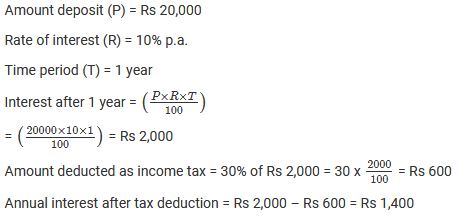```### Course Features

• 728 Video Lectures
• Revision Notes
• Previous Year Papers
• Mind Map
• Study Planner
• NCERT Solutions
• Discussion Forum
• Test paper with Video Solution